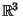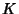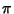## Polygonal Knot

A Knot equivalent to a Polygon in, also called a Tame Knot. For a polygonal knot, there exists a Plane such that the orthogonal projectionon it satisfies the following conditions:

1. The imagehas no multiple points other than a Finite number of double points.

2. The projections of the vertices ofare not double points of.
Such a projectionis called a regular knot projection.

References

Iyanaga, S. and Kawada, Y. (Eds.). Encyclopedic Dictionary of Mathematics. Cambridge, MA: MIT Press, p. 735, 1980.